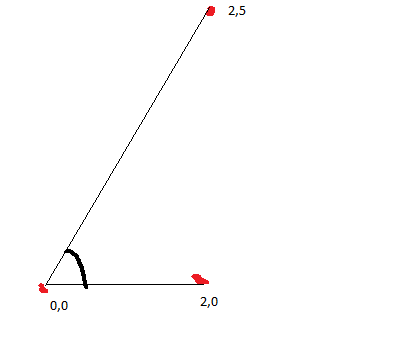# Vector2.Angle on "big" Vectors

Hello everybody.
So i am building an IA for a car game that i am building. The IA follow a sequence of waypoints so he can run in the track. My problem is that i need to calculate the curve of the waypoints so i can slow down on them. The method that i am using to do is calculate the angle between the current waypoint that i am going to and the next waypoint, so i can slow down before the curve starts. PS: I don’t know if this is the best way to do this, but i am starting to build this now so that was my first idea to start with. Anyway, so to calculate the angle i use Vector2.angle(actualWaypoint, nextWaypoint). The problem with that is that i can’t calculate the angle in the way that i want. Like at (2,0) and (2,5) and my car is at (0,0), i will have to do a 90º degree turn, but if i am at (300,0) and my points are at (302,0) and (302,5) the angle will be so small that the IA don’t know that he need to turn 90º degree as well.

Can someone help me figure this out? A better way to calculate the angle or an alternative?

Thank you very much and sorry for the wall text.

Look at what you did in your exampleYou basically moved the car from (0,0) to (300,0) and added the same (300,0) to both waypoints… Just subtract the car’s position vector from the waypoint vectors and you get the waypoints’ relative position compared to the car, and end up with the first equation you mentioned.

This however is not the solution to your steering problem I’m afraid. If you run this code

``````Debug.Log( Vector2.Angle(new Vector2(2,0), new Vector2(2,5)) );
``````

you can see it prints out `68.19859` degrees because that’s the angle between the direction vectors you gaveWhat’s even worse for you is that `Debug.Log( Vector2.Angle(new Vector2(2,0), new Vector2(2,-5)) );` prints out the same angle although you would probably want it to be negative since it’s an opposite turn.

What you probably want is the angle between vectors [car to wp1] and [wp1 to wp2] (or between vectors [wp0 to wp1] and [wp1 to wp2])

Mathf.Atan2() gives you the angle in positive or negative depending on direction, and something like this gives you the angle between 2 Vector2s (that go from A to B and B to C)

``````        Vector2 carPos = new Vector2(300,0);
Vector2 v1 = new Vector2(302,0);
Vector2 v2 = new Vector2(302,-5);

Vector2 diff1 = v1 - carPos;// direction from car to wp1
Vector2 diff2 = v2 - v1;	// direction from wp1 to wp2

float angle1 = Mathf.Atan2(diff1.y, diff1.x) * Mathf.Rad2Deg; // angle of direction 1
float angle2 = Mathf.Atan2(diff2.y, diff2.x) * Mathf.Rad2Deg; // angle of direction 2

Debug.Log( angle2 - angle1 ); // combined angle
``````

But rotations are tricky and you will probably still have problems with certain situations
(a la 180° + 2° = -178°)